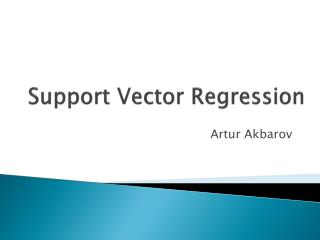DownloadDownload PresentationSupport Vector Regression

# Support Vector Regression

Download Presentation## Support Vector Regression

- - - - - - - - - - - - - - - - - - - - - - - - - - - E N D - - - - - - - - - - - - - - - - - - - - - - - - - - -
##### Presentation Transcript

1. Support Vector Regression ArturAkbarov

2. Paper A tutorial on support vector regression By Smola, A.J and Schölkopf, B. Statistics and Computing, 14, pp. 199-222, 2004

3. Why SVM? • SVM is applied in many different fields including bioinformatics, epidemiology, finance, economics etc. • Essentially, it can be applied wherever there is a problem of classification or prediction.

4. Formulation of SVR problem Source:http://www.saedsayad.com/support_vector_machine_reg.htm

5. Formulation of SVR problem Source:http://www.saedsayad.com/support_vector_machine_reg.htm

7. Parameter estimation Linear SVR: Non-linear SVR:

8. Kernels • Gaussian radial basis function: • Polynomial

9. Model over-fit

10. The solution of SVR • Model fitting: • Training set – fit the model • Validation set – predict using the fitted model, choose the model with minimum prediction error. • Model testing: • Test set – examine the prediction error (model performance, compare different prediction methods)

11. Splitting the data set • Training and validation sets: • Fixed split • Random split • Cross-validation • Split the data into n number of subsets, train on n-1 subsets, validate on the remaining subset, loop over all subsets. • Leave-one-out cross validation.

12. SVM library • LIBSVM – SVM library in different languages. • Weka – data mining tools. • R package - “e1071”

13. SVM in R • install.packages(“e1071”) • library(“e1071”) • model<-svm(data=D, formula=Y~X1+X2) • model<-svm(y=Y,x=X) • Y_fit<-predict(model, X) • Y_hat<-predict(model, X_new)

14. SVM in R • Other SVM parameters for the svm() function: • epsilon = 0.1 • cost = 1.0, which is C • kernel =“linear”, “polynomial”, “radial”, ”sigmoid”.

15. Tuning SVM parameters • best.svm() function uses grid search to find the optimal values for SVM parameters. • model<-best.svm(x=X, y=Y, tunecontrol=tune.control(cross=5), cost=c(1:10),epsilon=c(0.05,0.10))

16. Thank you for your attention